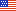An official website of the United States Government# NFS

Part Number: Part 1830

# PART 1830 - COST ACCOUNTING STANDARDS ADMINISTRATION

## Subpart 1830.70 - Facilities Capital Employed for Facilities in Use and For Facilities Under Construction

### 1830.7001-4 Postaward FCCOM applications.

(a) Interim billings based on costs incurred.

(1) The contractor may include FCCOM in cost reimbursement and progress payment invoices. To determine the amount that qualifies as cost incurred, multiply the incurred portions of the overhead pool allocation bases by the latest available cost of money factors. These FCCOM calculations are interim estimates subject to adjustment.

(2) As actual cost of money factors are finalized, use the new factors to calculate FCCOM for the next accounting period.

(b) Final settlements.

(1) Contract FCCOM for final cost determination or repricing is based on each year's final cost of money factors determined under CAS 414 and supported by separate Forms CASB-CMF.

(2) Separately compute contract FCCOM in a manner similar to yearly final overhead rates. As in overhead rates, include in the final settlement an adjustment from interim to final contract FCCOM. Do not adjust the contract estimated or target cost.

### 1830.7002-1 Definitions.

(a) Cost of money rate is either -

(1) The interest rate determined by the Secretary of the Treasury under Public Law 92-41 (85 Stat. 97); or

(2) The time-weighted average of such rates for each cost accounting period during which the capital asset is being constructed, fabricated, or developed.

(b) Representative investment is the calculated amount considered invested by the contractor during the cost accounting period to construct, fabricate, or develop the capital asset.

### 1830.7002-2 Cost of money calculations.

(a) The interest rate referenced in 1830.7002-1(a)

(1) is established semi-annually and published in the Federal Register during the fourth week of December and June.

(b) To calculate the time-weighted average interest rate referenced in 1830.7002-1(a)(2), multiply the rates in effect during the months of construction by the number of months each rate was in effect, and then divide the sum of the products by the total number of months.

### 1830.7002-3 Representative investment calculations.

(a) The calculation of the representative investment requires consideration of the rate or expenditure pattern of the costs to construct, fabricate, or develop a capital asset.

(b) If the majority of the costs were incurred toward the beginning, middle, or end of the cost accounting period, the contractor shall either:

(1) Determine a representative investment for the cost accounting period by calculating the average of the month-end balances for that cost accounting period; or

(2) Treat month-end balances as individual representative investments.

(c) If the costs were incurred in a fairly uniform expenditure pattern throughout the construction, fabrication, or development period, the contractor may either:

(1) Determine a representative investment for the cost accounting period by averaging the beginning and ending balances of the construction, fabrication, or development cost account for the cost accounting period; or

(2) Treat month-end balances as individual representative investments.

(d) The method chosen by the contractor to determine the representative investment amount may be different for each capital asset being constructed, fabricated, or developed, provided the method fits the expenditure pattern of the costs incurred.

### 1830.7002-4 Determining imputed cost of money.

(a) Determine the imputed cost of money for an asset under construction, fabrication, or development by applying a cost of money rate (see 1830.7002-2) to the representative investment (see 1830.7002-3).

(1) When a representative investment is determined for a cost accounting period in accordance with 1830.7002-3(b)(1) or 1830.7002-3(c)(1), the cost of money rate shall be the time-weighted average rate.

(2) When a monthly representative investment is used in accordance with 1830.7002-3(b)(2) or 1830.7002-3(c)(2), the cost of money rate shall be that in effect each month. Under this method, the FCCOM is determined monthly, and the total for the cost accounting period is the sum of the monthly calculations.

(b) The imputed cost of money will be capitalized only once in any cost accounting period, either at the end of the accounting period or the end of the construction, fabrication, or development period, whichever comes first.

(c) When the construction, fabrication, or development of an asset takes more than one accounting period, the cost of money capitalized for the first accounting period will be included in determining the representative investment for any future cost accounting periods.